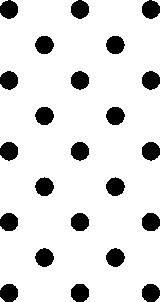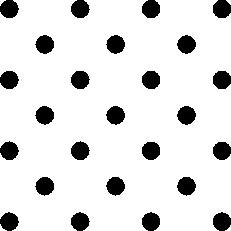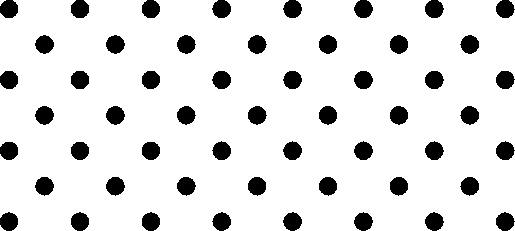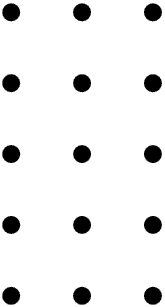#### You may also like### Prompt Cards

These two group activities use mathematical reasoning - one is numerical, one geometric.### Consecutive Numbers

An investigation involving adding and subtracting sets of consecutive numbers. Lots to find out, lots to explore.### Exploring Wild & Wonderful Number Patterns

EWWNP means Exploring Wild and Wonderful Number Patterns Created by Yourself! Investigate what happens if we create number patterns using some simple rules.

# Doplication

##### Age 7 to 11Challenge Level

While I'm sitting in front of the computer I also have a dice and it is showing a $5$. Rather like this:-This is quite a popular arrangement for this number.

Going around I see that some people wear ties or dresses that  are very colourful and full of lovely patterns. The patterns may be made up of teddy bears, rockets, pink-panthers, flowers and all sorts of other things. However there arrangement - if I represent each item just as a black dot then the pattern is usually :-The arrangements usually sit quite well into a rectangular arrangement. So we might call this last one a $3$ by $5$ or a $5$ by $3$ arrangement. Squares are just special rectangles and so we could have a $4$ by $4$ :-When we have the $3$ by $5$ we can count the dots and see that we have $23$ altogether. Now $3$ times $5$ IS NOT $23$. So we cannot write it as:- $3 x 5= 23$

So we (You) will have to invent another name instead of times and a sign to go with it. Perhaps (because it is convenient on the computer) you may choose "?" BUT you can choose anything that you like!

Now I can write:-

$3 ? 5 = 23$

and when I look at the $2$ by $2$ and the $4$ by $4$ I can write them as:-

$2 ? 2 = 5$ and $4 ? 4 = 25$

If you now look at a bigger arrangement that follows the same kind of pattern you'll find various ways of counting up the number of dots needed.

So let's look at this one $8 ? 4$Count them up, calculate, or do whatever so that you find out how many there are altogether and we have:- $8 ? 4 = 53$. Invent some more questions of your own and work out the answers.

Now if this were an ordinary thing like multiplication, which you'd use in an ordinary rectangle like:-You probably would know the answer straight away or you'd be able to work it out from things that you knew before. When people learn multiplication tables they often write them out in a big table. So you could explore what happens if you try a similar idea with doing "?" instead of multipliation and make a ?Table instead of a TimesTable.